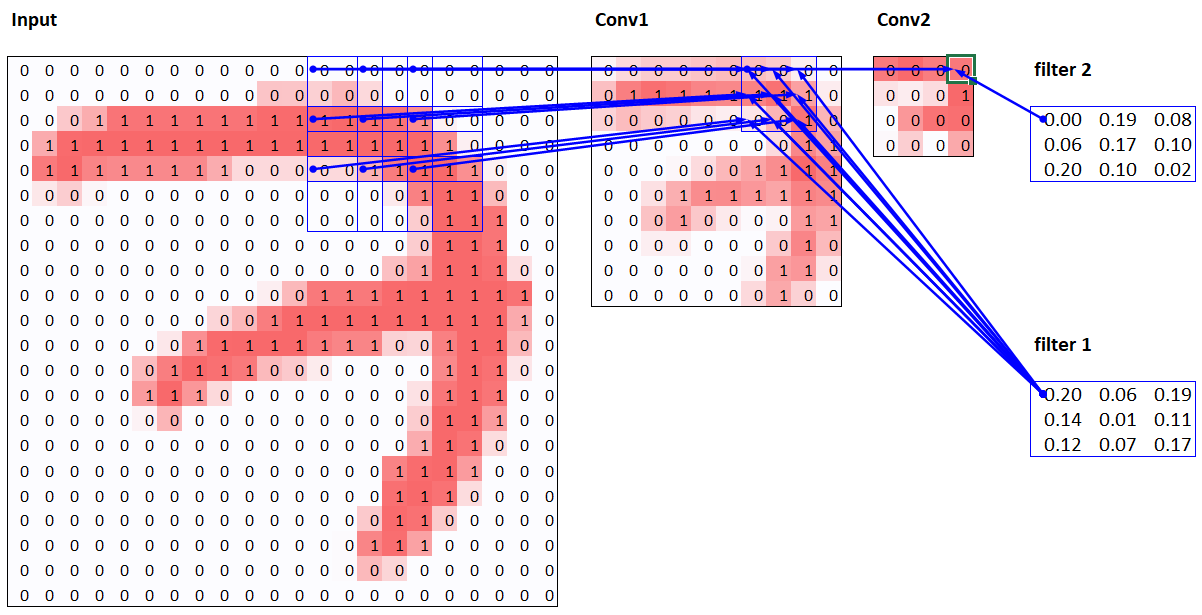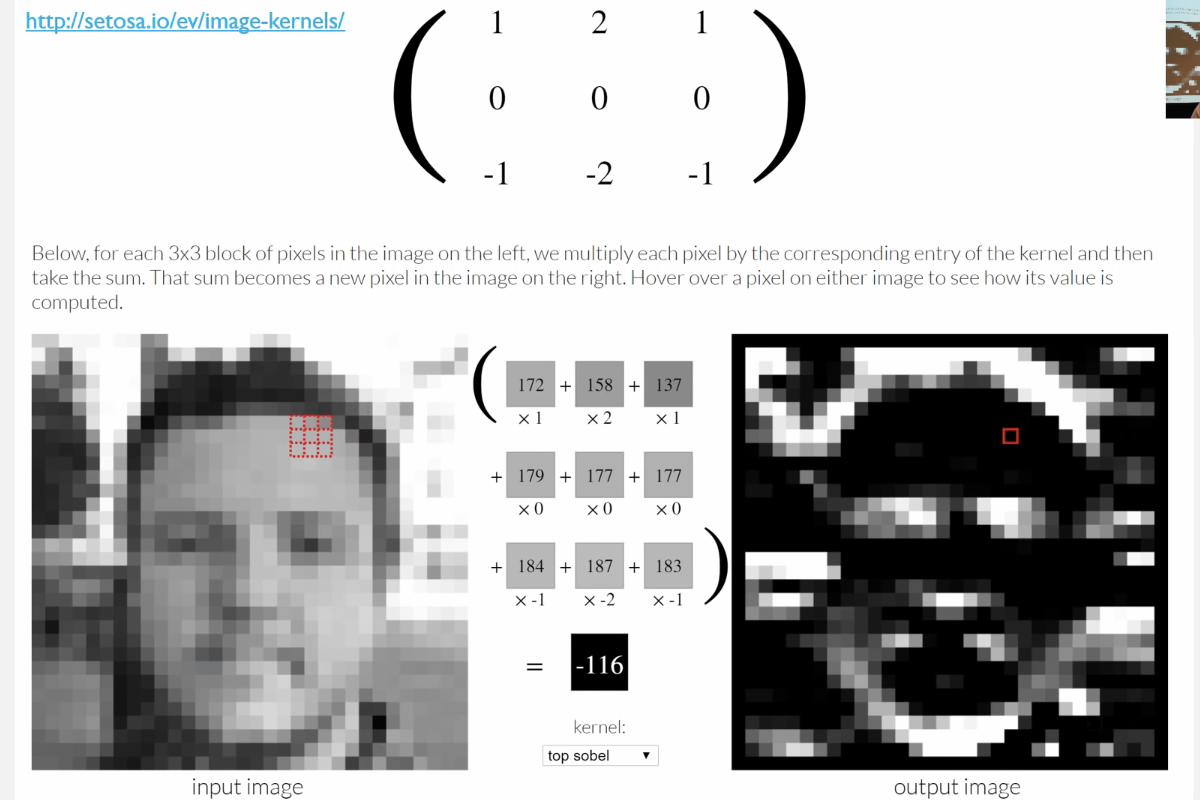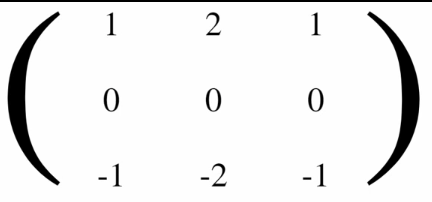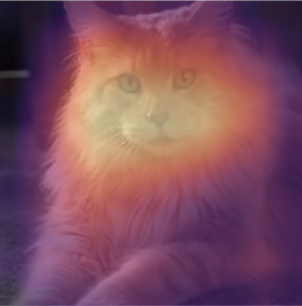# Part1 lesson6 note | fastai 2019 course-v3

Apr 15, 2020 · 8 mins read[v1] Thu, 16 Apr 2020

[v2] Thu, 23 Apr 2020

# Lesson 06

Will find official notes here

## Rossmann(Tabular)

• Tabular data: be careful on Categorical variable vs Continuous variable.
• if datatype is int, fastai think it is classification, not a regression.
• Root mean square percentage error. as loss function.
• When you assign the y_range, it’s better to assign little bit more than actual maximum. > because it’s sigmoid.
• Intermediate layers, which is weight matrix is 1) 1000, and 2) 500 -> which means our parameter would be 500*1000.
learn.model


### What is dropout and embedding dropout?

Nitish Srivastava, Dropout: A Simple way to prevent Neural Networks from Overfitting

• you can dropout with p value, make it specified to specific layer, or make it applied to all the layers.
• Pytorch code 1) bernoulli, which decides whether you will hold it? 2) and divide the noise value depends on noise value. so noise became 2 or remain 0.
• According to pytorch code, We do change at training time, but we do nothing at test time. and this means you don’t have to do anything special with inference time.’
• TODO: find at forums what is inference time - Related to NVIDIA, GPU.
• Embedding dropout is just a dropout.
• It’s different between continuous variable and embedding layer. TODO Still can’t understand. why embedding dropout is effective. or,… in need.
• Let’s delete at random, some of the results of the embedding.
• and It worked well especially at Kaggle

### Batch Normalization

• The key was multiplicative bias gamma and additive bias beta
• Explain
• Let $$\hat{y} = f(w_1, w_2, w_3, … , x)$$ , loss = MSE , Then y_range should be between 1 and 5
• And Activation function ends with -1 -> +1
• To mitigate this problem, we can add another parameter, like $$w_n$$
• But there’re so much interactions in the process so just re-scale the output.

### Momentum parameter at BatchNorm1d

• Different from momentum like in optimization.
• This momentum is Exponentially weighted moving average of the mean, instead of deviation.
• If this is small number: mean standard deviation would be less from mini_batch to mini_batch » less regularization effect. (If this is large number, variation would be greater from mini_batch to mini_batch » more regularization effect)
• TODO: can’t sure, but i understand, this is not about how to update parameter but about how much reflect previous value when scale and shift

Q. Preference between batchnorm and the other regularizations(drop out, weight decay)

A. Nope, always try and see the results

## lesson6-pets-more.ipynb

### Data Augmentation

• Last reg
• get_transforms has lots of params (even not yet learned all) -> check documentation
• Remember you can implement all the doc contents bc it’s made from nbdev
• TODO: try this!!
• Essence of data augmentation is you should maintain the label, while somewhat making sense.
• ex) tilt, because it’s optically sensible, you can always change the angle of the data view.
• zeros, border, and reflection but always reflection works most of the time, so that is the default

### Convolutional Kernel(What is convolution?)

• Will make heat_map from scratch, which means the parts convolution focuses on• http://setosa.io/ev/image-kernels/
• javascript thing
• How convolution works
• Kernel. which does element-wise multiplication, and sum them up
• so it has on pixel less at borders -> so it uses padding, and fastai uses reflection as said.
• why this Kernel(matrix) helps catching horizontal edge side?• CNN from different viewpoints
• output of pixel is results from different linear equations.
• If you connect this with represents of neural network nodes, you can see that the specific inp nodes connected with specific out nodes.
• Summarize: cnn does 1) matmul some of the elements are always zero 2) same weight for every row, which is called weight time? weight..?, 1:18:50 (picture)

#### Further lowdown

• Because generally image has 3 channels, we need rank 3 kernel.
• And do multiply with all channel output is one pixel.(draw by your self)
• but this kernel will catch one feature, like horizontal, so that we make more kernel so that output becomes (h * w * kernel)
• And that kernel come to channel
• Stride 2 conv: with 3 by 3 kernel, stride 2 conv -> (h/2 * w/2 * kernel) 1
• skip or jump over input pixel
• to protect from memory out of control
learn.model
learn.summary()


TODO: understand yourself the blocks of conv-kernel:

• Usually use big kernel size at first layer (will study this at part2) 2
• Bottom&right highlighting kernel, since that parts are positive numbers
k = tensor([
[0.  ,-5/3,1],
[-5/3,-5/3,1],
[1.  ,1   ,1],
]).expand(1,3,3,3)/6

• Why divided by 6, when doing expand? : forum answer
• torch.tensor.expand: for memory efficient, because we should do RGB
• We do not make separate kernel, but make rank 4 kernel
• 4d tensor is just stacked kernel
• t[None].shape create new unit axis, and why? we make this -> it should move unit of batch, not one size image.

### Average pooling, feature

• suppose our pre-trained model results in size of 11 by 11 by 512  and my classification task has 37 classes
• take the first face of channel, which is 11 by 11 and mean it, so that make rank 2 tensor, 512 by 1
• and make 2d matrix, which is 512 by 37 and multiply so that we can get 37 by 1 matrix.
• Feature, at convolution block
• So, when we transfer-learning without unfreeze, every element of last matrix (512 by 1) should represent(or could catch) each feature.

### Heatmap, Hookhook_output(model) -> acts -> avg_acts

• if we average the block with axis=feature, result of matrix(11 by 11) depicts how activated was that area? -> it is heatmap, avg_acts
• and acts comes from hook, which is more advanced pytorch feature.
• hook into pytorch machine itself, and run any arbitrary Pytorch code
• Why this is cool?: Normally it gives set of outputs of forward pass, but we can interrupt and hook the forward pass.
• Also can store the output of the convolutional part of the model, which is before avg_pooling
• Thinking back when we do cut off after the conv part.
• but with fast.ai the original convolutional part of the model would be the first thing in the model, specifically could be given from learn.model.eval()
• And this is gotten from hooked_output and having hooked the output, we can pass our x_minibatch to output.
• Not directly, but with normalized, minibatch, put on to the gpu
• one_item() function do it, when we have one data TODO: this is assignment do it yourself without one_item function
• and .cuda() put it on gpu
• you should print out very often the shape of tensor, and try think why.

(personal) Further research

• Yes, as notes of official course, the ConvNN have become more and more important for other ML model but computer vision. (see Convolutional Block Attention Module relationship paper) and nlp is much more fall behind of computer vision at modern deep learning research, less augmentation method, resource optimization, performance(even not comparable, they both has standard task), … SO How about enhance nlp model using this (kind of) relationship?

Footnote

1. what is conv1d, conv2d, conv3d?

2. why use comparatively huge kernel at first layer?